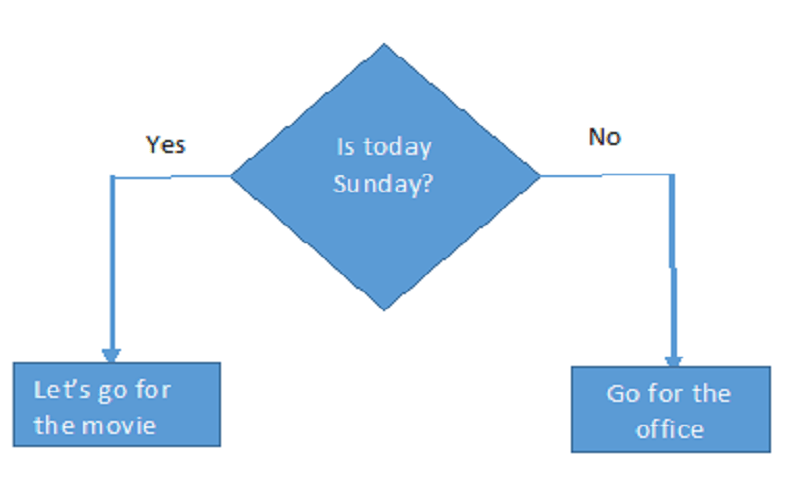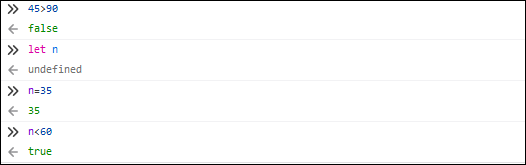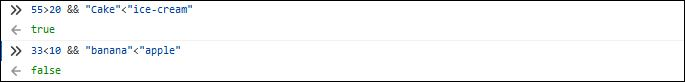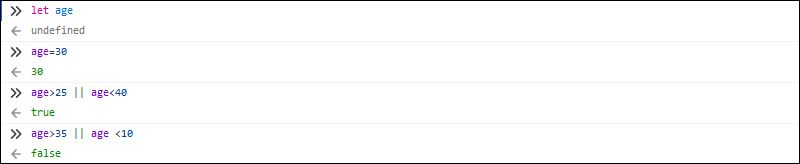# FahmidasClassroom

Learn by easy steps### Conditional statement

Conditional statement is an essential part of any programming language. It is used to execute one or more statements based on particular condition. Each condition works on a Boolean value that can be true or false. Like other programming languages, if, else if, else and switch statements are used in JavaScript to perform the conditional tasks. This tutorial will help you to learn the uses of if-else statement in JavaScript.

The following topics will be covered in this tutorial:

• Comparison Operator
• Logical Operator
• If, else if and else statements
• Ternary operator
• Switch-case statement

Before going to the next part of this tutorial, you can check the following diagram to get the basic concept on conditional statement.Exercise:

No exercise is assigned for this part of the tutorial.

### Comparison Operator:

You have to learn about the comparison operators before learning the uses of conditional statement. These operators are used to compare values in conditional statement. The following comparison operators are used in JavaScript to compare values.

 Operator Description == Return true when the values are equal. === Return true when the values and data types are equal. != Return true when the values are not equal. !== Return true when the values or data types are not equal. < Return true when the left value is less than the right value. <= Return true when the left value is less than or equal to the right value. > Return true when the left value is greater than the right value. >= Return true when the left value is greater than or equal to the right value.

### Comparing Numeric values:### Compare String values:Exercise:

Declare a variable named myvar by using ‘let’ command and assign the value, 70. Compare the value of myvar with 55 for equivalence.

### Logical Operator:

If two or more conditions are required to check for executing any task, then logical operators are used to define the conditions. The following operators are mainly used as logical operators in Javascript.

 Operator Description && It is known as AND operator and it returns true when all conditions are true. || It is known as OR operator and it returns true when any of the conditions is true. ! It is known as NOT operator and it returns the opposite Boolean value of the condition. That means if the condition returns true then NOT operator will make it false or vice-versa.

Using logical AND:Using logical OR:Using logical NOT:Exercise:

Declare three variables, username and department by using ‘let’ command. Assign two string values into these variables.

1. Write a condition using logical AND that will return true when the username is “admin” and the department is “Sales”.
2. Write a condition using logical OR that will return true when the username is “admin” or the department is “HR”

### If, if-else and if-elseif-else statements:

Simple ‘if‘ statement is used for executing any task when the condition is true. ‘if-else’ statement is used to execute one task for true condition and execute another task for false condition. ‘If-elseif-else’ statement is used to define multiple conditions and if the first condition returns false then it will check the second condition, if the second condition returns false then the third condition will check and so on. If all conditions return false, then the task of else block will execute. The examples of these three types of statements are given below.

If statement:

``````
var book='JQuery';
if (book == 'HTML')
if (book == 'JQuery' || book == 'HTML')

``````

If-else Statement:

``````
var book=prompt("Enter the book name","")
if (book == 'HTML')
document.write('Learning HTML5');
else
document.write('Design website using HTML and CSS');
``````

If-elseif-else statement:

``````
var book=prompt("Enter the book name","")
if (book == 'HTML')
document.write('Learning HTML5');
else if(book == "CSS")
document.write('Design website using HTML and CSS');
else if(book == "Javascript")
document.write('Design website using HTML and Javascript');
else
document.write('Web Design Fundamentals');
``````

Exercise:

Write a javascript code to take a number from 0 to 100 using prompt() and write if-elseif-else conditions to print “A+” if the number is more than or equal to 80, “A” if the number is less than 80 but greater than or equal to 75, “A-” if the number is less than 75 and greater than or equal to 70 and “F” for other value.

### Ternary Operator:

Ternary operator is used as an alternative of if-else statement. ‘?’ and ‘:’ are used to define ternary operator. When the condition returns true then the left-side statement of ‘:’ executes otherwise the right-side statement of ‘:’ executes.

Conditional statement using ternary operator:

``````
var message=(name == "") ? "You have to type your full name" : "Welcome, \$name";;
``````

Exercise:

Write a Javascript code to take a weekday name from the user and check the input value using ternary operator. If the value is “Sunday” then print “Holiday” and for other value print “Working day”.

### Switch-case Statement:

Switch-case is an alternative of if-elseif-else statement. Each condition is defined by ‘case’ statement and if the value matches then a particular condition(s) will execute. ‘break’ statement is used in each case statement to terminate from the switch-case block.

``````
var n=prompt("Enter a number between 1 and 4","")
var x=10,y=15;
switch(n) {
case "1":
break;
case "2":
document.write("Subtraction="+(y-x))
break;
case "3":
document.write("Multiplication="+(x*y))
break;
case "4":
document.write("Division="+(y/x))
break;
default:
document.write("Invalid Entry")
}

``````

Exercise:

Write a javascript code to take the month name as input and define condition using switch case to check the month. if the month name is July then print “Summer Vacation”, if the month name is December then print “Winter Vacation” and print “No vacation” for other month.

### Conclusion

All types of conditional statements of javascript are explained in this tutorial by using various examples. This tutorial will help the new javascript user to use conditional statement in javascript.